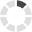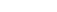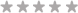### Site InformationLoading... Please wait...
• My Account

# FIN 370 Week 3 Assignment Question and Problem Sets

\$15.00
Quantity:## Product Description

Ch. 9: Questions 7 & 8 (Questions and Problems section)

7.   Calculating IRR [LO5] A firm evaluates all of its projects by applying the IRR rule. If the required return is 14 percent, should the firm accept the following project?

8.   Calculating NPV [LO1] For the cash flows in the previous problem, suppose the firm uses the NPV decision rule. At a required return of 11 percent, should the firm accept this project? What if the required return is 24 percent?

Ch. 10: Questions 3 & 13 (Questions and Problems section)

3.   Calculating Projected Net Income [LO1] A proposed new investment has projected sales of \$635,000. Variable costs are 44 percent of sales, and fixed costs are \$193,000; depreciation is \$54,000. Prepare a pro forma income statement assuming a tax rate of 35 percent. What is the projected net income?

13.   Project Evaluation [LO1] Dog Up! Franks is looking at a new sausage system with an installed cost of \$540,000. This cost will be depreciated straight-line to zero over the project’s five-year life, at the end of which the sausage system can be scrapped for \$80,000. The sausage system will save the firm \$170,000 per year in pretax operating costs, and the system requires an initial investment in net working capital of \$29,000. If the tax rate is 34 percent and the discount rate is 10 percent, what is the NPV of this project?

Ch. 11: Questions 1 & 7 (Questions and Problems section)

1.  Calculating Costs and Break-Even [LO3] Night Shades, Inc. (NSI), manufactures biotech sunglasses. The variable materials cost is \$9.64 per unit, and the variable labor cost is \$8.63 per unit.

a. What is the variable cost per unit?

b. Suppose NSI incurs fixed costs of \$915,000 during a year in which total production is 215,000 units. What are the total costs for the year?

c. If the selling price is \$39.99 per unit, does NSI break even on a cash basis? If depreciation is \$465,000 per year, what is the accounting break-even point?

7.  Calculating Break-Even [LO3] In each of the following cases, calculate the accounting break-even and the cash break-even points. Ignore any tax effects in calculating the cash break-even.

## Product Reviews

This product hasn't received any reviews yet. Be the first to review this product!

## Customers also viewed

• \$15.00• \$15.00• \$15.00• \$15.00• \$15.00## Related Products

Click the button below to add the FIN 370 Week 3 Assignment Question and Problem Sets to your wish list.